### Adding an Import terrains from several files node

This node is available for the Pro edition only.

This node composes several input bitmap files to make a single terrain. The size and gray scale of each image is treated in the same way as for the Import terrain node and uses the same operation for file management, but instead of entering a file name, a formula is entered, which is used to determine the name of each file.

To add a node, right-click in the Graph Editor and select Create Node  > Import  > Import terrain from several files.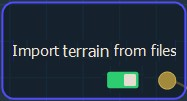Double click on the node to open its parameters: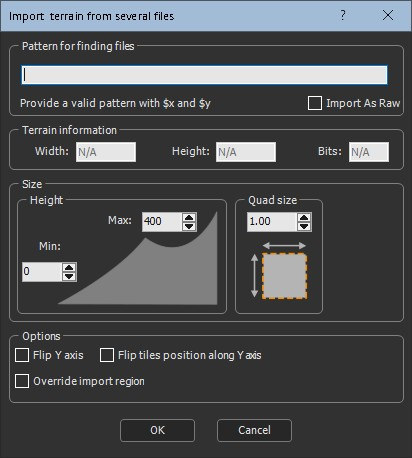### Importing a terrain from several files

To import a terrain from several files, set the following parameters:

• Pattern for finding files: This is the formula used to retrieve the files to load. The naming convention is important because the node looks for all the files with the same name and aligns them on a grid where the first number is the X axis and the second one is the Y axis.

See Explanation about the formula for a detailed description about the formula.

To import them into Instant Terra, browse to the folder where your files are located. Copy and paste the path in the parameters dialog.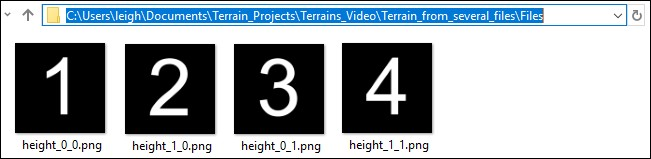Replace the first number, which represents the X axis, by \$x and the second number, which represents the Y axis, by \$y.

Only nodes with a single channel, 8 bits, 16 bits, or 32 bits, are supported.
- For 8 bits, the values are between 0 and 255.
- For 16 bits, the values are between 0 and 65'535.
- For 32 bits, the values are floating values: for a terrain, this is the height of the terrain.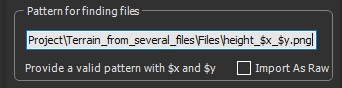The files are imported to form one terrain and each one is aligned in the correct position.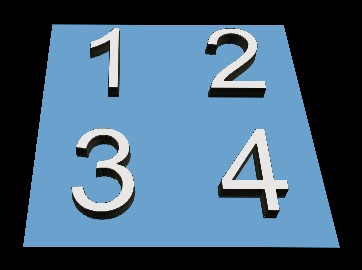• Terrain information: Lists the Width and Height of the terrain. These parameters are non-editable.
• Quad size: Sets the terrain quad size. In the example below, the quad size is increased by 50%.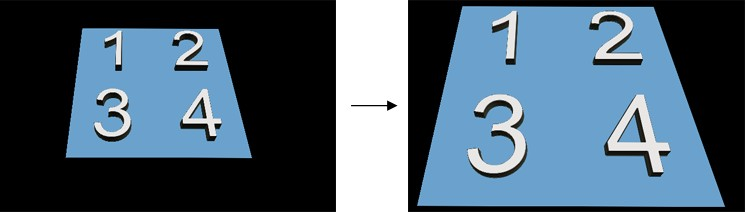• Height: Sets the minimum and maximum height of the terrain. In the example below, the Max height is increased.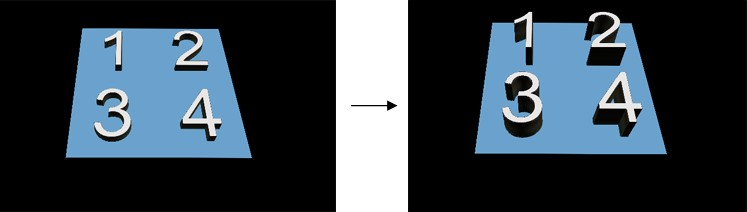• Flip Y axis: Check the box to flip the terrain around the Y axis.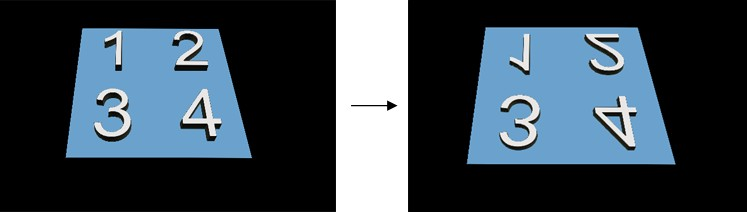• Flip tiles position along Y axis: Check the box to flip the position of the tiles along the Y axis. The files with the smallest Y axis are at the bottom, and the files with the largest Y index are at the top. The X axis does not change.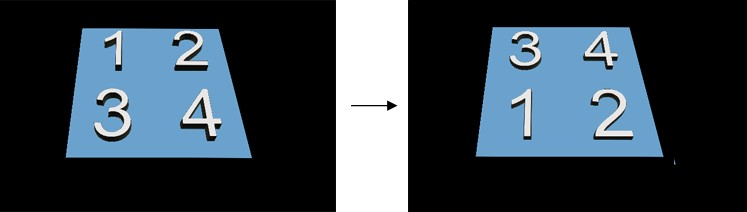By default, the part corresponding to the first file (for example, file (1, 1)) is at the top left of the field and the last file (for example (4, 3)) is at the bottom right of the field. This means that we go down when the index Y increases.

• Override import region: Check the box to override the import region if you want to specify the files to load; otherwise, Instant Terra detects the files to load automatically. Set the Region to load parameters to specify the files to load with the corresponding coordinates. For example, if you have 12 files, load only the files with a min and max Y coordinate of 1, a max X coordinate of 1, and a min Y coordinate of 2.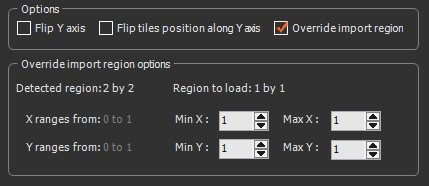• Click OK to load the terrain files.

### Explanation about the formula

For the imported files, the node:

• Replaces \$y by 0, and finds the largest value of \$x that exists for the files, i.e. input_\$x_0.bmp. This determines one dimension of the grid of files, called X.
• Replaces \$x by 0, and finds the largest value of \$y that exists for the files, i.e. input_0_\$y.bmp. This determines the other dimension of the grid of files, called Y.
• The node iterates on \$y and \$x and calculates the image size, i.e. width and height.

Each file is loaded, and forms the corresponding part of the final terrain.

The replacement rules in the file names (or directory) are:

• \$x is replaced by: 0, 1, ..., 10, 11, ...
• \$0x is replaced by: 00, 01, ..., 10, 11, ..., 100, ...
• \$00x is replaced by: 000, 001,..., 010, 011,..., 100,...
• \$000x is replaced by: 0000, 0001,..., 0010, 0011,..., 0100,..., 1000
• \$0000x is replaced by: 00000, 00001,..., 00010, 00011,..., 00100,..., 01000,..., 10000,...
• \$00000x is replaced by: 000000, 000001,..., 000010, 000011,..., 000100,..., 001000,...
• Ditto for \$y, \$0y, \$00y, \$000y, \$0000y, and \$00000y.
• The expressions above can be used:
• In the name of the file and in the directory name.
• In one or more copies.
• Mixed (for both \$X and \$Y).

For example:

Expression Product names
terrain/\$0x/\$0y/file.bmp terrain/00/00/file.bmp
terrain/01/00/file.bmp
terrain/10/24/file.bmp
terrain/\$0x/\$0y/file_\$x_\$y.bmp terrain/00/00/file_0_0.bmp
terrain/01/00/file_1_0.bmp
terrain/10/24/file_10_24.bmp

## Parameters

Parameter Use
File name Name of the file to load
Quad size Size of the terrain quads
Terrain information Shows the width, height, and bits of the terrain
Flip Y axis Flips the terrain around the Y axis
Flip tiles position along Y axis Flips the position of the tiles along the Y axis
Override import region Overrides the import region to specify the files to load